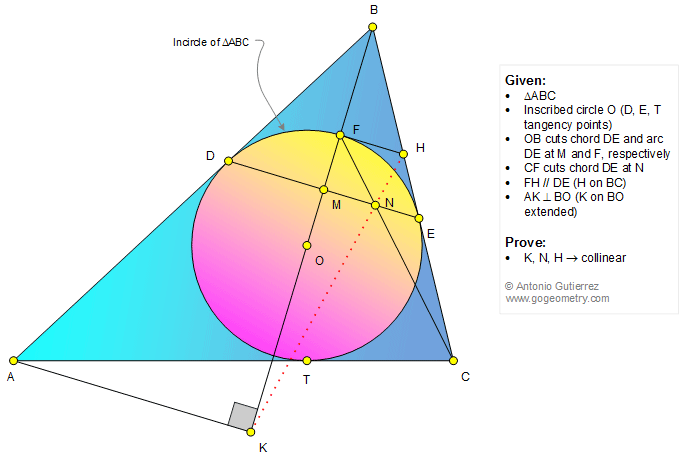#Geometry Problem 1320: Triangle, Incircle, Tangent, Chord, Circle, Parallel, Perpendicular, Collinearity.

 < PREVIOUS PROBLEM  |  NEXT PROBLEM > The figure shows a triangle ABC with the inscribed circle O (D, E, and T are the tangency points). OB cuts chord DE and arc DE at M and F, respectively. CF cuts chord DE at N. FH is parallel to DE (H on BC) and AK is perpendicular to BO (K on BO extended). Prove that points K, N, and H are collinear.See also: Sketch of problem 1320.

 Home | Geometry | Problems | All Problems | Open Problems | Visual Index | 1311-1320 | Triangle | Circle | Incircle | Tangent line | Collinear Points | Parallel lines | View or Post a solution | by Antonio Gutierrez